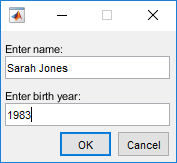# assignin

## 语法

``assignin(ws,var,val)``

## 说明

``assignin(ws,var,val)` 将值 `val` 赋给工作区 `ws` 中的变量 `var`。例如，`assignin('base','x',42)` 将值 42 赋予 MATLAB® 基础工作区中的变量 `x`。如果需要计算 `val`，MATLAB 会在调用 `assignin` 的函数中进行计算，而不是在 `ws` 指定的工作区进行计算。如果 `val` 是函数句柄，则它在调用 `assignin` 的函数中必须是可计算的。`assignin` 函数对于以下任务很有用：将数据从函数导出至基础工作区。从函数中更改调用方函数的工作区中定义的变量值。例如，您可以在调用函数参数列表中更改变量的值。`

## 示例

```function c = myAdd(a,b) c = a+b; str = sprintf('%s called with %d,%d (%s)',mfilename,a,b,char(datetime)); assignin('base','fcnStatus',str) end```

`n = myAdd(2,3)`
```n = 5```

`fcnStatus`
```fcnStatus = 'myAdd called with 2,3 (17-Nov-2017 14:56:14)'```

```function mydialog prompt = {'Enter name:','Enter birth year:'}; answer = inputdlg(prompt); n = answer{1}; birthyear = str2double(answer{2}); a = 2050-birthyear; assignin('base','name',n); assignin('base','age2050',a); end```

`mydialog````function updateAge(a) validateattributes(a,{'numeric'},{'scalar'}) fprintf('\tYour age: %d\n',a) localfcn fprintf('\tYour updated age: %d\n',a) end function localfcn assignin('caller','a',42) end```

`updateAge(37)`
``` Your age: 37 Your updated age: 42```

```function m = minRand(n) assignfh A = fh(n) m = min(A(:)); end function assignfh fh = @(dim)rand(dim); assignin('caller','fh',fh) end```

`m = minRand(2)`
```A = 0.3486 0.1423 0.0419 0.0766 m = 0.0419```

`minRand` 示例相似，`assignfh2` 局部函数将 `fh` 分配给 `minRand2` 的工作区。`assignfh2` 函数使用名为 `rand` 的变量覆盖其工作区中的 `rand` 函数并创建函数句柄。这种行为与匿名函数一致 - 函数句柄是使用创建函数时可用的变量创建的。因此，在 `minRand2` 中对函数句柄进行计算后，会使用 `n``assignfh2` 中定义的 `rand` 数组进行索引。

```function m = minRand2(n) assignfh2(n) A = fh(n) m = min(A(:)); end function assignfh2(n) rand = 13*ones(n); fh = @(dim)rand(dim); assignin('caller','fh',fh) end```

`m = minRand2(2)`
```A = 13 m = 13```

## 输入参数

• 您从命令提示符下调用函数，而主函数调用 `assignin`

• 您从命令提示符下调用 `assignin`

## 提示

• `assignin` 函数不会为数组的特定元素赋值。因此，`var` 不能包含数组索引。以下代码将导致错误。

```X = 1:8; assignin('base','X(3:5)',-1); ```

要为数组的特定元素赋值，请使用 `evalin` 函数。

```evalin('base','X(3:5) = -1') ```